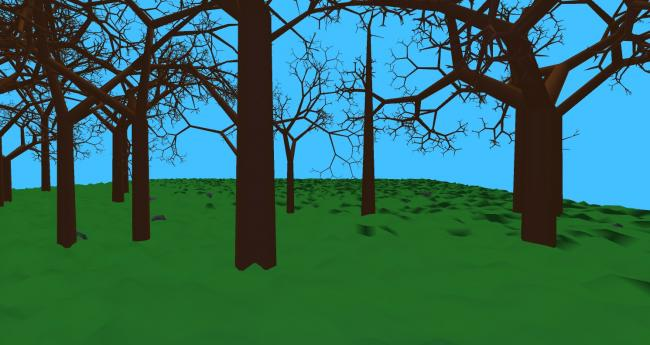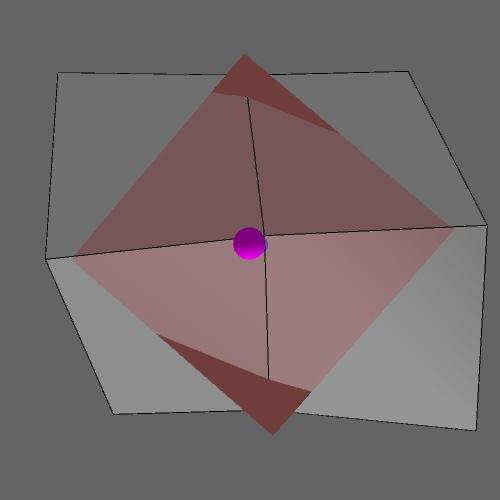#### Howdy, Stranger!

We are about to switch to a new forum software. Until then we have removed the registration on this forum.

# Trying to set the normal of uneven ground:shading gets messed up when far away

edited May 2016

So I'm trying to set the custom normal for my ground and I think I did something wrong with the formula. Here is the code for the ground:

``````PeasyCam cam;
Ground ground;
PImage grassTexture;

void setup(){
size(displayWidth-100, displayHeight-100, OPENGL);
ground=new Ground(new PVector(0,200,0), 12000, 15, 6000, 50, grassTexture);

cam = new PeasyCam(this, 100);
cam.setMinimumDistance(50);
cam.setMaximumDistance(500);
}

void draw(){
background(0,172,255);
ambientLight(120, 120, 120);  //regular light that reaches everywhere

lightFalloff(1, .001, .0001);
//lightSpecular(5, 5, 5); //gives tiny glow to ground in darkness, might take out when I make ground with more segments
directionalLight(170, 170, 170, .5, 1, 0);  //creates shadow

ground.render();
}

class Ground {

PVector pos;
float gWidth, gHeight, gLength;
float squareDim;

PImage t;
float[][] pointHeight;
ArrayList<ArrayList<PVector>> normals;

PShape ground;

Ground(PVector pos, float gWidth, float gHeight, float gLength, float squareDim, PImage t) {
this.pos=pos;
this.gWidth=gWidth;
this.gHeight=gHeight;
this.gLength=gLength;
this.squareDim=squareDim;
this.t=t;
pointHeight=new float[(int)(gWidth/squareDim)][(int)(gLength/squareDim)];
for (int i=0; i<(int)(gLength/squareDim); i++) {
for (int j=0; j<(int)(gWidth/squareDim); j++) {
pointHeight[j][i]=pos.y+random(-gHeight, gHeight);
}
}
ground=createShape(GROUP);
assignNormals();
draw();
}

void assignNormals(){
normals=new ArrayList<ArrayList<PVector>>();
float leftTop, leftMiddle, leftBottom, middleTop, middleMiddle, middleBottom, rightTop, rightMiddle, rightBottom;
for (int i=0; i<gLength/squareDim; i++) {
ArrayList<PVector> rowNormals=new ArrayList<PVector>();
middleMiddle=pointHeight[i];
leftMiddle=middleMiddle+random(-gHeight, gHeight);

if (i>0) {
middleTop=pointHeight[i-1];
leftTop=middleTop+random(-gHeight, gHeight);
} else {
middleTop=middleMiddle+random(-gHeight, gHeight);
leftTop=(middleTop+middleMiddle)/2+random(-gHeight, gHeight);
}
if (i<(int)gLength/squareDim-1) {
middleBottom=pointHeight[i+1];
leftBottom=middleBottom+random(-gHeight, gHeight);
} else {
middleBottom=middleMiddle+random(-gHeight, gHeight);
leftBottom=(middleBottom+middleMiddle)/2+random(-gHeight, gHeight);
}
for (int j=0; j<gWidth/squareDim; j++) {
if (j>gWidth/squareDim-2) {
println("last in column");
rightMiddle=middleMiddle+random(-gHeight, gHeight);
rightTop=(rightMiddle+middleTop)/2+random(-gHeight, gHeight);
rightBottom=(rightMiddle+middleBottom)/2+random(-gHeight, gHeight);
} else {
//rightMiddle=middleMiddle+random(-gHeight, gHeight);
rightMiddle=pointHeight[j+1][i];
if (i>0) {
//rightTop=rightMiddle+random(-gHeight, gHeight);
rightTop=pointHeight[j+1][i-1];
} else {
rightTop=rightMiddle+random(-gHeight, gHeight);
}
if (i<(int)gLength/squareDim-1) {
//rightBottom=rightMiddle+random(-gHeight, gHeight);
rightBottom=pointHeight[j+1][i+1];
} else {
rightBottom=rightMiddle+random(-gHeight, gHeight);
}
}
float middle=(leftTop+leftMiddle+leftBottom+middleTop+middleBottom+rightTop+rightMiddle+rightBottom)/8;
float left=(leftTop+leftMiddle+leftBottom+middleTop/2+middleBottom/2)/4;
float top=(leftTop+middleTop+rightTop+leftMiddle/2+rightMiddle/2)/4;

//is something wrong in here??????
////////////////////////////////////////////////////////////////////////////////////
PVector normal=new PVector(-(left-middle), -squareDim/2, -(top-middle));
normal.normalize();
//////////////////////////////////////////////////////////////////////////////////////

leftTop=middleTop;
leftMiddle=middleMiddle;
leftBottom=middleBottom;

middleTop=rightTop;
middleMiddle=rightMiddle;
middleBottom=rightBottom;
}
}
}

void render() {
shape(ground);
}

}

void Hill(PVector loc) {
PVector placement=PVector.sub(loc, pos);
placement.div(squareDim);
}

void draw() {
PShape strip;
for (int i=0; i<(int)(gLength/squareDim-1); i++) {
strip=createShape(GROUP);
ArrayList<PVector> top=new ArrayList<PVector>();
ArrayList<PVector> bottom=new ArrayList<PVector>();
top=normals.get(i);
bottom=normals.get(i+1);
for (int j=0; j<(int)(gWidth/squareDim-1); j++) {
PVector normal1=top.get(j);
PVector normal2=bottom.get(j);
PVector normal3=top.get(j+1);
PVector normal4=bottom.get(j+1);
float point1=pointHeight[j][i];
float point2=pointHeight[j][i+1];
float point3=pointHeight[j+1][i];
float point4=pointHeight[j+1][i+1];

}
}
}
}
``````Does anyone know how to fix this? Is it the normal that is facing into the ground?

Tagged:

• edited July 2014

The dark spots show up as light spots underneath the ground

• it's easy enough to draw the normals so you can see if there's anything wrong. might need to make the tiles larger though.

using a cross product is the usual way of calculating normals. there may be a PVector method for it which takes two PVectors as input, outputs the normal. yes, here: http://processing.org/reference/PVector_cross_.html

http://en.wikipedia.org/wiki/Cross_product

(couldn't run the above, my processing2 is old and didn't know about createShape(GROUP))

•I have been testing it. The normal is right as far as I can see

• not even sure what i'm looking at there.

grey quads are what? the surface? pink quad is what? i take it the pink dot is the end of the normal, pointing up, and we are looking down.

• grey quads are the surface and the pink is the plane of the average orientation of all the points. purple/pink is the normal and the small blue sphere behind is the average height of all the points

• I think that the problem is caused by the "light" hitting vertexes that are at the top and bouncing straight up rather than towards the camera. As far as I know you cannot change the diffuse value of individual vertexes so I don't think that it can be fixed. That is why moving towards the dark bits will make them disappear because the "light" will now hit your eye.

• Instead of creating a lot of individual shapes in a group, I think you should create a ground that is ONE SINGLE shape. Your current code is kind of inefficient because you send the same texture to the GPU over and over and you send each quad as a separate (albeit group) shape instead of one single shape, which is quite possible for this kind of geometry.

Also, if you want more control over the lighting, you can do that in the vertex or fragment shader. Then you have all the control you want over the lighting! See for example this code where custom lighting calculation are in the vertex shader: https://github.com/AmnonOwed/P5_CanTut_GeometryTexturesShaders2B8/tree/master/GLSL_HeightmapNoise